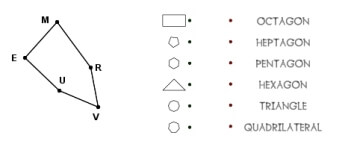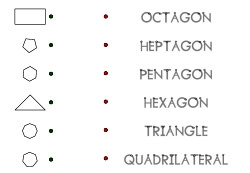Worksheets and No Prep Teaching Resources Math Worksheets Geometry PrintablesPolygons Worksheets, Printables, and Lesson Plans

Kindergarten Geometry - Introduction to Drawing Some Simple Polygons

Shapes

Matching PolygonsMatching Polygons

Drawing Shapes Challenge Lesson - Dot Game

Which Polygon?
Which polygon?

Classify Polygons
Classify polygons

Area and Perimeter of Polygons
Find the perimeter of each figure
Find the perimeter of each figure using a ruler
Perimeter: Find the value of n
Find the area of each figure
Find the value of n
Find the area and perimeter of each rectangle (no graphics)
Find the perimeter of a complex polygon (composed of 2 rectangles)
Find the perimeter of a complex polygon (composed of 3 rectangles)
Find the perimeter of a complex polygon (composed of 4 rectangles)
Find the area and perimeter of a complex polygon (composed of 2 rectangles)
Find the area and perimeter of a complex polygon (composed of 3 rectangles)
Find the area and perimeter of a complex polygon (composed of 4 rectangles)
Area and Perimeter Mixed Review (Grade 4)

Part 2
Area of a parallelogram (lengths are whole numbers)
Area of a parallelogram (lengths are decimals)
Area of a parallelogram (lengths are fractions)
Area of a triangle (lengths are whole numbers)
Area of a triangle (lengths are decimals)
Area of a triangle (lengths are fractions)

Angles of Polygons
Angles of Triangles
Angles of Polygons